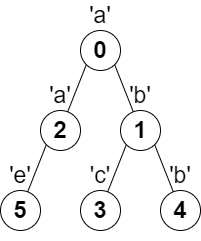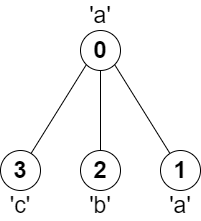2246. 相邻字符不同的最长路径

# 2246. 相邻字符不同的最长路径#

• 标签：树、深度优先搜索、图、拓扑排序、数组、字符串
• 难度：困难

## 题目大意 #

• $n == parent.length == s.length$。
• $1 \le n \le 10^5$。
• 对所有 $i \ge 1$ ，$0 \le parent[i] \le n - 1$ 均成立。
• $parent == -1$。
• $parent$ 表示一棵有效的树。
• $s$ 仅由小写英文字母组成。

• 示例 1：1 2 3 4  输入：parent = [-1,0,0,1,1,2], s = "abacbe" 输出：3 解释：任意一对相邻节点字符都不同的最长路径是：0 -> 1 -> 3 。该路径的长度是 3 ，所以返回 3。 可以证明不存在满足上述条件且比 3 更长的路径。 
• 示例 2：1 2 3  输入：parent = [-1,0,0,0], s = "aabc" 输出：3 解释：任意一对相邻节点字符都不同的最长路径是：2 -> 0 -> 3 。该路径的长度为 3 ，所以返回 3。 

## 解题思路 #

### 思路 1：树形 DP + 深度优先搜索 #

1. 如果其最长路径经过根节点 $u$，则 $最长路径长度 = 某子树中的最长路径长度 + 另一子树中的最长路径长度 + 1$。
2. 如果其最长路径不经过根节点 $u$，则 $最长路径长度 = 某个子树中的最长路径长度$。

1. 先计算出从相邻节点 $v$ 出发的最长路径长度 $v\underline{}len$。
2. 更新维护全局最长路径长度为 $self.ans = max(self.ans, \quad u\underline{}len + v\underline{}len + 1)$。
3. 更新维护当前节点 $u$ 的最长路径长度为 $u\underline{}len = max(u\underline{}len, \quad v\underline{}len + 1)$。

### 思路 1：代码 #

  1 2 3 4 5 6 7 8 9 10 11 12 13 14 15 16 17 18 19 20 21 22 23 24  class Solution: def __init__(self): self.ans = 0 def dfs(self, graph, s, u): u_len = 0 # u 节点的最大路径长度 for v in graph[u]: # 遍历 u 节点的相邻节点 v_len = self.dfs(graph, s, v) # 相邻节点的最大路径长度 if s[u] != s[v]: # 相邻节点字符不同 self.ans = max(self.ans, u_len + v_len + 1) # 维护最大路径长度 u_len = max(u_len, v_len + 1) # 更新 u 节点的最大路径长度 return u_len # 返回 u 节点的最大路径长度 def longestPath(self, parent: List[int], s: str) -> int: size = len(parent) # 根据 parent 数组，建立有向图 graph = [[] for _ in range(size)] for i in range(1, size): graph[parent[i]].append(i) self.dfs(graph, s, 0) return self.ans + 1 

### 思路 1：复杂度分析 #

• 时间复杂度：$O(n)$，其中 $n$ 是树的节点数目。
• 空间复杂度：$O(n)$。